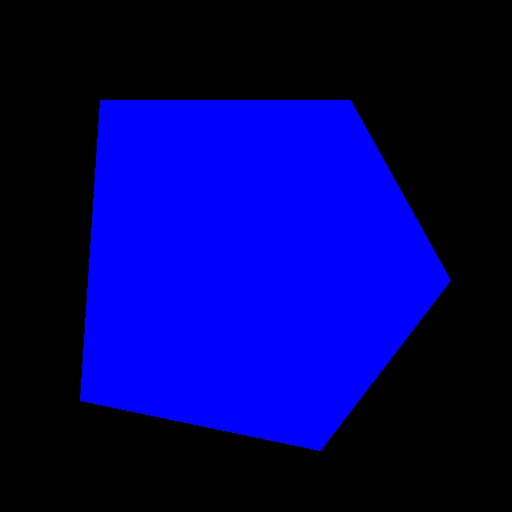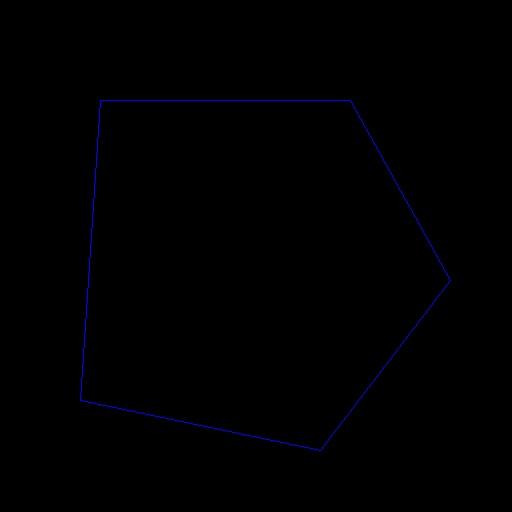Hong_Youth

V1

2021/10/08阅读：303主题：默认主题

# OpenCV-Python绘制多边形和选择随机颜色

## 绘制多边形

• `cv.polylines()`多边形绘制
需要传入画布、轮廓点、是否闭合、绘制颜色、线条粗细、线条类型、顶点坐标小数位。

• `cv.fillPoly()`多边形填充
需要传入画布、轮廓点、填充颜色、线条类型、顶点坐标小数位。

• `drawContours()`既可以填充也可以绘制多边形
需要传入画布（三通道画布才能显示轮廓）、轮廓点、绘制轮廓数（-1表示绘制其中的所有轮廓）、颜色、轮廓粗细（-1表示填充）。

``# -*-coding:utf-8-*-"""File Name: image_shape_painting.pyProgram IDE: PyCharmDate: 21:12Create File By Author: Hong"""import cv2 as cvimport numpy as npdef polygon_drawing():    canvas = np.zeros((512, 512, 3), dtype=np.uint8)    # 定义多边形的顶点    pts = np.array([[100, 100], [350, 100], [450, 280], [320, 450], [80, 400]], dtype=np.int32)    # 多边形绘制    # cv.polylines(canvas, [pts], True, (0, 0, 255), 2, 8, 0)    # 多边形填充    # cv.fillPoly(canvas, [pts], (255, 0, 255), 8, 0)    # 既可以填充也可以绘制形状, thickness为时绘制形状，-1时填充形状    # 可以添加多个轮廓，用,号隔开，比如[pts1, pts2, ...]    cv.drawContours(canvas, [pts], -1, (255, 0, 0), thickness=-1)    cv.imshow('polyline', canvas)    cv.waitKey(0)    cv.destroyAllWindows()    if __name__ == '__main__':    polygon_drawing()``## 随机颜色和随机数

1. `np.random.randn()`函数

`np.random.randn(d0,d1,d2……dn)`
1）当函数括号内没有参数时，则返回一个浮点数；
2）当函数括号内有一个参数时，则返回秩为1的数组，不能表示向量和矩阵；
3）当函数括号内有两个及以上参数时，则返回对应维度的数组，能表示向量或矩阵；
4）`np.random.standard_normal()`函数与`np.random.randn()`类似，但是`np.random.standard_normal()`的输入参数为元组（tuple）；
5)`np.random.randn()`的输入通常为整数，但是如果为浮点数，则会自动直接截断转换为整数；
6）通过该函数可以返回一个或一组服从标准正态分布的随机样本值。

1. `np.random.rand()`函数

`np.random.rand(d0,d1,d2……dn)`

1）通过本函数可以返回一个或一组服从 “0~1”均匀分布 的随机样本值。随机样本取值范围是[0,1)，不包括1。

1. np.random.randint()函数

`numpy.random.randint(low, high=None, size=None, dtype=’l’)`

low—–为最小值
high—-为最大值
size—–为数组维度大小
dtype—为数据类型，默认的数据类型是`np.int`

``"""File Name: image_shape_painting.pyProgram IDE: PyCharmDate: 21:12Create File By Author: Hong"""import cv2 as cvimport numpy as npdef random_color():    img = np.zeros((300, 300, 3), dtype=np.uint8)    cv.imshow('img', img)    while True:        # 设置随机位置        xx = np.random.randint(0, 300, 2, dtype=np.int32)        yy = np.random.randint(0, 300, 2, dtype=np.int32)        # 设置随机颜色，可以用于目标检测画不同目标边界框的随机颜色        bgr = np.random.randint(0, 255, 3, dtype=np.int32)        print(bgr, bgr, bgr)        # 画直线，将每个bgr分量int转换以下，不然被认为不是数字，出错。        cv.line(img, (xx, yy), (xx, yy), (int(bgr), int(bgr), int(bgr)), 1, 8, 0)        cv.imshow('line', img)        c = cv.waitKey(1)        if c == 27:            break    cv.destroyAllWindows()if __name__ == '__main__':    random_color()``V1

AI改变生活